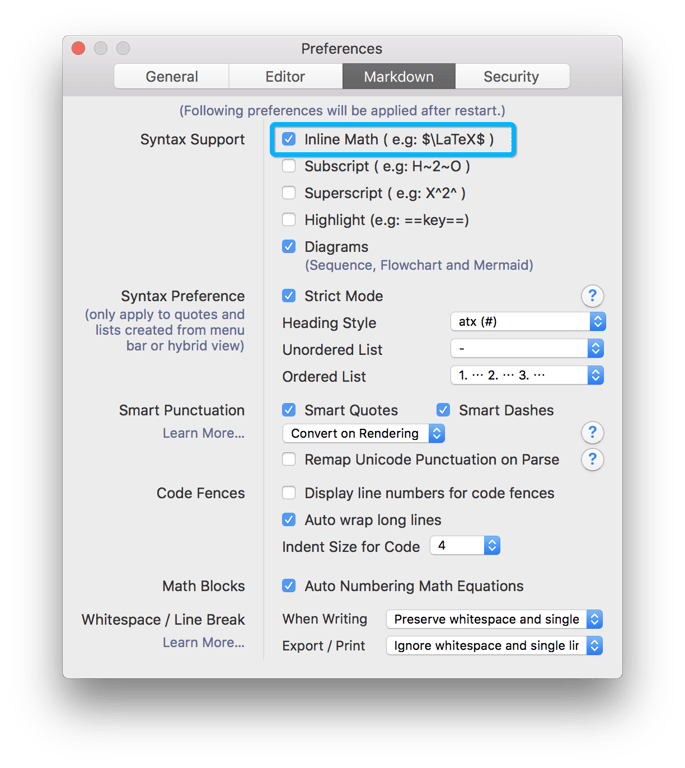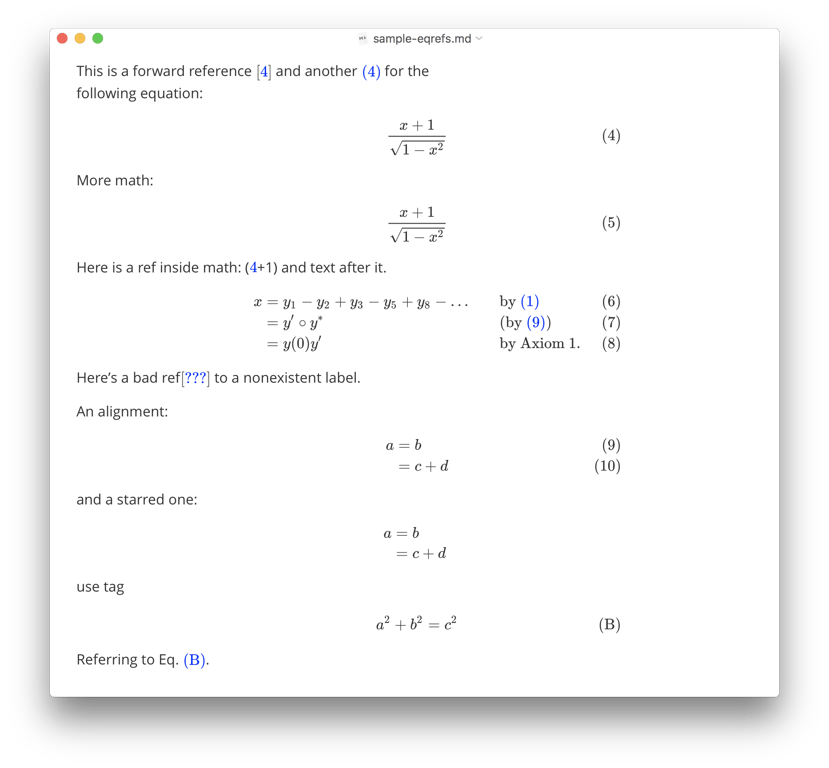# 数学和学术功能 #

Typora 支持使用 Tex/LaTeX 语法渲染普通数学。渲染过程是由 MathJax (opens new window) 支持。

## 数学块（显示数学） #

\begin{align*}
y = y(x,t) &= A e^{i\theta} \\
&= A (\cos \theta + i \sin \theta) \\
&= A (\cos(kx - \omega t) + i \sin(kx - \omega t)) \\
&= A\cos(kx - \omega t) + i A\sin(kx - \omega t)  \\
&= A\cos \Big(\frac{2\pi}{\lambda}x - \frac{2\pi v}{\lambda} t \Big) + i A\sin \Big(\frac{2\pi}{\lambda}x - \frac{2\pi v}{\lambda} t \Big)  \\
&= A\cos \frac{2\pi}{\lambda} (x - v t) + i A\sin \frac{2\pi}{\lambda} (x - v t)
\end{align*}
$$ 将被渲染为 (opens new window) 在 typora 中，你可以只按 $$Return 键来输入一个数学块，在输入模式下，使用上/下箭头键或 Command/ Ctrl + Return 键来完成编辑，或直接点击✓ 按钮，或其他地方。

## Inline Math ### Typora 中可用的 TeX 命令 #

$$\def\bold#1 \bold{this\ is\ now\ bold}$$


## Chemistry Expressions #

Typora 有内置的 mhchem (opens new window) 扩展，可以用来渲染化学表达式，你可以像这样使用。

$\ce{CH4 + 2$\left( \ce{O2 + 79/21 N2} \right)$}$


## 相互参照 #

Here is a labeled equation:

$$x+1\over\sqrt{1-x^2}\label{ref1}\tag{1}$$

This is a reference : $\ref{ref1}$


## 自动编号 #

Typora 支持自动编号的数学块。要打开这个功能，请打开偏好设置，并在 "Markdown "部分启用 "自动编号数学方程"。## 故障排除 #

### displaylines #

\displaylines{x+y\\y+z}